# MathMath games that will teach you about mathematics, numbers and counting. Practice the basic number perception with an abacus, or practice the four rules of arithmetic. You will learn math in a joyful way by playing these games. Here we have collected all the games on the subject Math. The games suit preschool, elementary school and all the way up to high school. The games may be sorted by popularity, target group, release date or name.
0%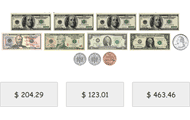## Count money

• Money
• Counting
• Mental calculation
Look at the notes and coins and add up the sum. Practicing basic knowledge in how to count money with increasing difficulty. This knowledge game uses all valid denominations of the Swedish krona, U.S.
0%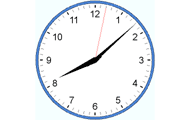## Tell time in English

• Clock
• Analog clock
• Numbers
Practice analog clock. Practice the basics such as full hours, half-hours, quarters and minutes. Watch the three clocks and choose the clock that match the requested time.
0%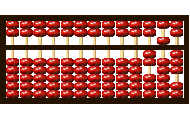## Abacus - Basics

• Logical thinking
• Mental calculation
• Abacus
Learn to count on an abacus online. In order to be able to count on an abacus you have to master the basics. In this game you train the values of the beads, the importance of the beam and the rods.
0%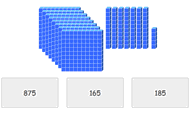## Place value with base ten blocks

• Counting
• Mental calculation
Look at the picture and count the hundreds. Then count the number of tens and after that the ones. Sum up and click on the correct number. Practice counting and improve your perception of numbers in t
0%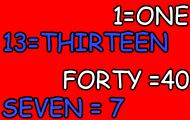## Writing numbers in words

• Numbers
• Digits
• Words
• Write
Practice how numbers are written in words. In English and both numbers to words and words to numbers.
0%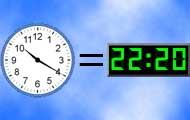## Match clocks

• Clock
• Analog clock
• Digital clock
• Numbers
Match clocks that can be both analog and digital. Practice telling time with both types of clocks.
0%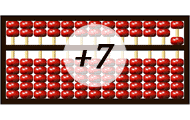• Logical thinking
• Abacus
Learn to count addition on an abacus. In order to be able to perform addition on an abacus you need to master the abacus basics. In this game you practice to carry extra digits when adding numbers.
0%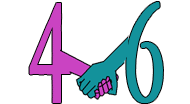## Number bonds

• Numbers
• Mental calculation
• Number bonds
Try to find the number that is a number bond to another number and adds up to the given sum. Practice part-part-whole relationships to the numbers 0-20 and facilitates the important mental calculation
0%## Minecraft math

• Multiplication
• Division
• Subtraction
• Problem solving
Count with Minecraft! You will need to multiply, divide and subtract.
0%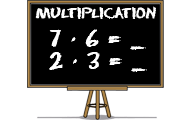## Multiplication

• Numbers
• Multiplication
• Mental calculation
Learn multiplication with this math game for students. Find the product of the factors in all times tables.
0%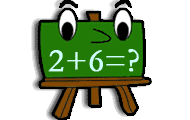• Numbers
• Mental calculation
Practice addition in math game with several levels of increasing difficulty. Add two numbers and find the correct sum.
0%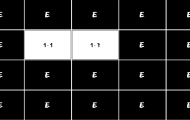## Multiplication memory

• Multiplication
• Times tables
• Mental arithmetic
• Memory training
Practice multiplication with times tables in this memory game where you should match an expression with the right product.
0%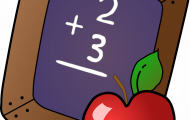• Fluency
This game will help students build fluency when adding within 10.
0%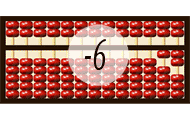## Abacus - Subtraction

• Logical thinking
• Subtraction
• Abacus
Practice subtraction on the abacus. In this game you practice to borrow when performing math calculation with subtraction. Please play the other abacus games before playing this game for better unders
0%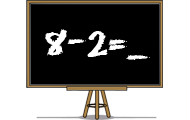## Subtraction

• Numbers
• Subtraction
• Mental calculation
Practice subtraction or minus in a skill training game. Find the difference between two numbers in different levels in this math game.
0%## Sums of two one-digit numbers

• 1-digit number
students will be adding one-digit numbers in this game.
0%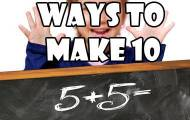## Ways to make 10

• Number bonds
• Make 10
These friends of ten activities really help students learn ways to make ten in a fun and hands on way.
0%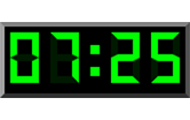## Digital clock in English

• Clock
• Digital clock
• Numbers
Practice the digital clock with 24 hours. Read a written time and find the corresponding time among the three digital clocks.
0%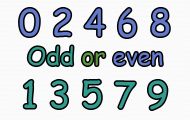## Odd or even numbers

• Even
• Odd
• Numbers
Here you get to practice recognizing even and odd numbers.
0%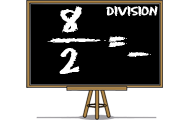## Division

• Numbers
• Division
• Mental calculation
Practice division, ie dividing two numbers. Find the quotients of the numerators divided by the denominators in this math game.
0%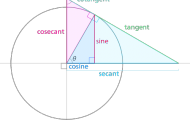## Trigonometry Vocabulary

• Trigonometry
• Functions
• Vocabulary
Practice the six trigonometric functions and other trigonometry definitions.
0%## Balance the Equations

• Equalities
• Balancing equations
• Subtraction
Use your addition and subtraction skills to balance the equations and win the game!
0%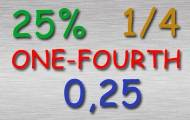## Convert fractions, percentages and decimals

• Fractions
• Percents
• Decimals
• Conversion
Practice convert a number between fraction, percentage and decimal. Includes the ten steps from a whole of one-tenth.
0%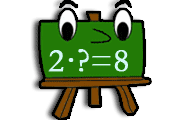## Find the factor

• Factor
• Multiplication
• Product
Try to find the requested factors in this game of multiplication. You get a factor and a product and your task is to find the missing product.
0%## Volume Concentration

• Volume
• Formula
• 3D figures
Match up the corresponding 3D figure to its volume formula.## Create your own Math game

• Quickly
• Easy
• Free of charge
Missing a game? Are you a teacher, expert or educating in the subject Math? Please feel free to create your own educational math game with your own questions for a lesson, practice or test. Choose from several different game templates.
Weekly top list
1.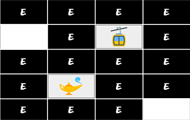Brain training
2.Brain training
3.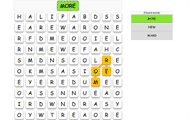English
4.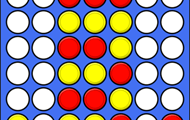Brain training
5.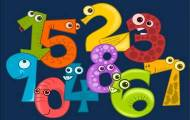Spanish